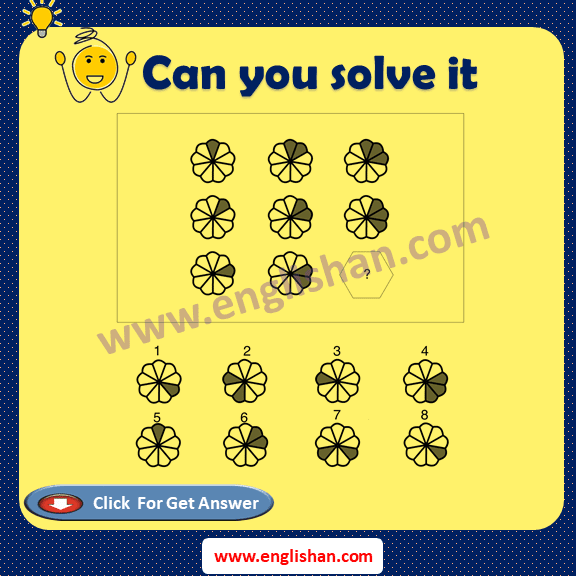WhatsApp Groups

# IQ Test Questions with Answer | Free IQ Test Oline 2020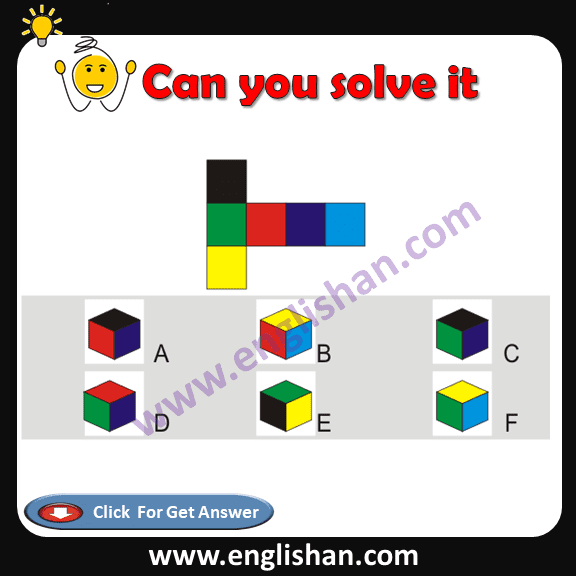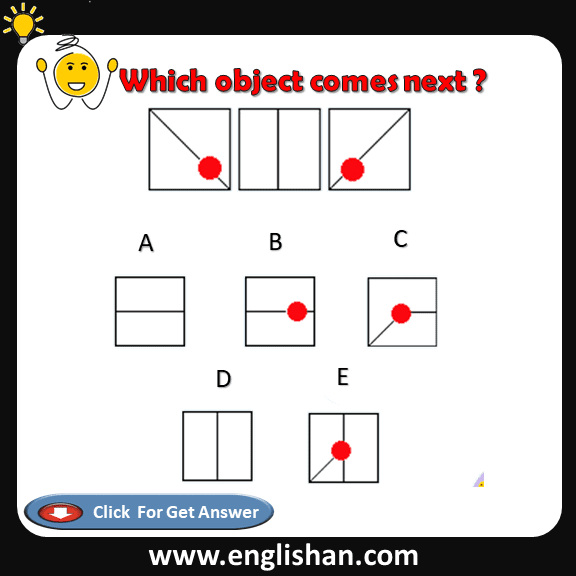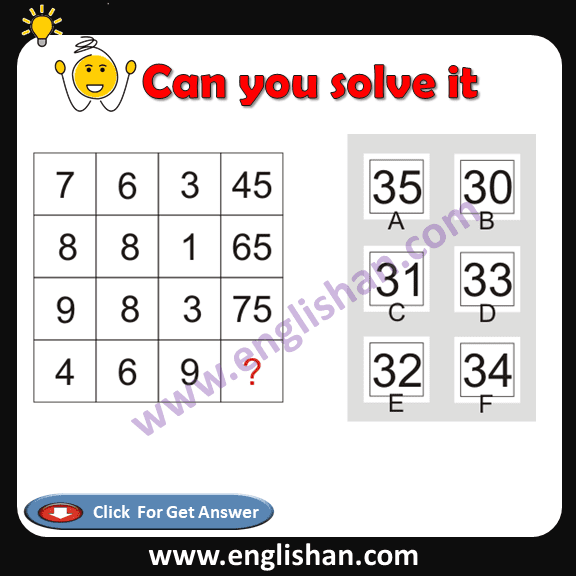Explanation: (7 x 6) + 3 = 45 | (8 x 8) + 1 = 65 |

(9 x 8) + 3 = 75

(4 x 6) + 9 = 33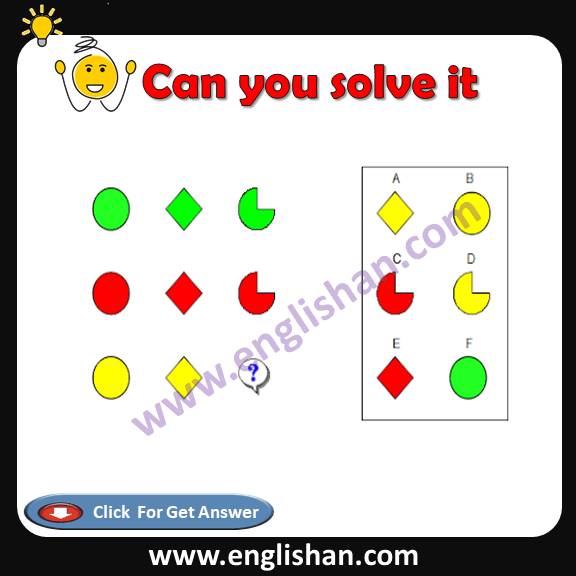Correct Answer : D